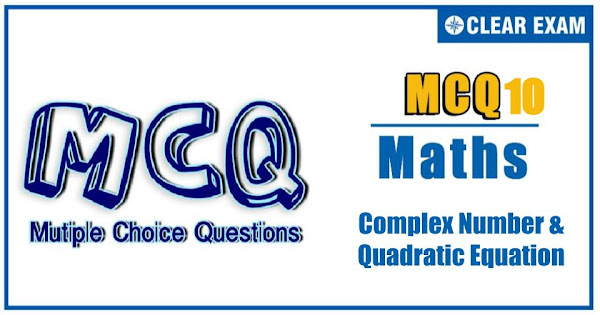## [LATEST]\$type=sticky\$show=home\$rm=0\$va=0\$count=4\$va=0

Complex numbers and quadratic equations is a segment of maths that deals with crucial theorems and concepts along with various formulae. It comprises of linear and quadratic equations along with roots related to the complex number's set (known as complex roots)..

Q1.  The number of integral values of a for which the quadratic equation (x+a)(x+1991)+1=0 has integral roots are
•  3
•  0
•  1
•  2
Solution
(d)
(x+a)(x+1991)+1=0
⇒(x+a)(x+1991)=-1
⇒(x+a)=1 and x+1991=-1
⇒a=1993
or x+a=-1 and x+1991=1 ⇒a=1989
Q2. Let z and ω be two complex numbers such that |z| ≤ 1, |ω| ≤ 1 and |z - iω|= |z - i¯ω|=2 then z equals
•  1 or i
•  i or –i
•  1 or -1
•  i or -1
Solution
(c)
We have, 2 = |z-iω| ≤ |z| + |ω| (∵|z1+z2 |≤|z1 |+|z2 |)
∴|z| + |ω| ≥ 2 (i)
But given that |z|≤1 and |ω|≤1. Hence
⇒|z| + |ω|≤2 (ii)
From (i) and (ii),
|z|=|ω|=1
Also, |z+iω|=|z-i¯ω|
⇒|z-(-iω)|=|z-i¯ω|
Hence, z lies on perpendicular bisector of the line segment joining (-iω) and (i¯ω), which is a real axis, as (-iω) and (i¯ω) are conjugate to each other. For z,Im(z)=0. If z= x, then
|z| ≤ 1 ⇒ x2≤1
⇒ -1 ≤ x ≤ 1

Q3. z1 and z2 lie on a circle with centre at the origin. The point of intersection z3 of the tangents at z1 and z2 is given by
•  1/2 (¯z1+¯z2)
•  (2z1 z2 )/( z1+z2 )
•  1/2 ( 1/z1 + 1/z2 )
•  (z1+z2)/(¯z1 ¯z2 )

Q4. If α and β be the roots of the equation x2+px-1/(2p2)=0 where p∈R. Then the minimum value of α44 is
•  2√2
•  2 - √2
•  2
•  2 + √2
Q5. If α,β are the roots of ax2 + c = bx, then the equation (a+ cy)2 = b2 y in y has the roots
•  αβ(-1)(-1) β
•  α(-2)(-2)
•  α(-1)(-1)
•  α22
Q6. If (cos⁡θ+i sin⁡θ )(cos2θ+i sin⁡2θ )⋯(cos⁡nθ+i sin⁡nθ ) = 1, then the value of θ is, m∈N
•  4mπ
•  2mπ/( n(n+1) )
• 4mπ/(n(n+1))
•  mπ/( n(n + 1))
Q7. The roots of the cubic equation(z + ab)3=a3, such that a ≠ 0, represent the vertices of a triangle of sides of length
•  1/√3 |ab|
•  √3|a|
•  √3|b|
•  |a|
Q8. A quadratic equation whose product of roots x1 and x2 is equal to 4 and satisfying the relation x1/(x1-1) + x2/( x2-1)=2 is
•  x2-2x+4=0
•  x2+2x+4=0
•  x2+4x+4=0
•  x2-4x+4=0
Q9.  If the equation cot4⁡x -2cosec2x + a2 = 0 has at least one solution then, sum of all possible integral values of a is equal to
•  4
•  3
•  2
•  0
Q10. The number of irrational roots of the equation 4x/(x2+x+3)+5x/(x2-5x+3)=-3/2 is
•  4
•  0
•  1
• 2
Solution
(d)
Here, x=0 is not a root. Divide both the numerator and denominator by x and put x+3/x=y to obtain
4/(y+1)+5/(y-5)=-3/2⇒y=-5,3
x+3/x=-5 has two irrational roots and x+3/x=3 has imaginary roots#### Written by: AUTHORNAME

AUTHORDESCRIPTION## Want to know more

Please fill in the details below:

## Latest NEET Articles\$type=three\$c=3\$author=hide\$comment=hide\$rm=hide\$date=hide\$snippet=hide

Name

ltr
item
BEST NEET COACHING CENTER | BEST IIT JEE COACHING INSTITUTE | BEST NEET & IIT JEE COACHING: Complex Numbers and Quadratic Equations-Quiz-10